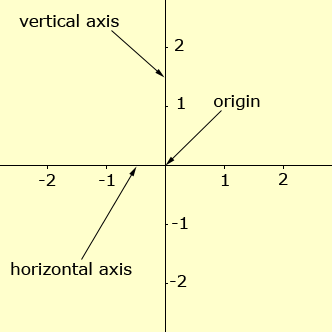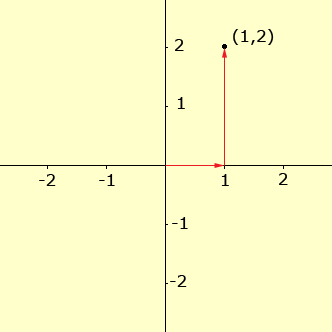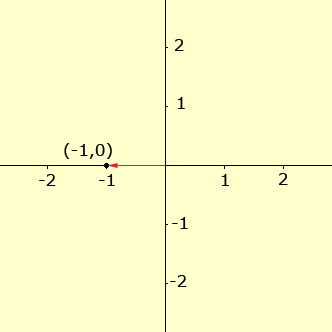Subject: plotting co-ordinates on a number plane Name: Victor Who are you: Student (Middle) I need to plot co-ordinates (0,2), (2,-2), (-2,0), and (0,0) on a number or co-ordinates plane, I am not sure how to do this, and get lost after the first point, can you please help? Hi Victor, The number plane as you call it is the plane with two perpendicular lines on it which I am going to call the horizontal axis and the vertical axis. Your textbook and teacher may have different names for these axis. The point where the axes intersect is called the origin. Also each of the axes have a scale on them with 0 at the origin. O the horizontal axis positive is to the right and on the vertical axis positive is upwards.You can think of the co-ordinates of of a point, written (first_co-ordinate, second_co-ordinate) as instructions to get from the origin to the point. The first_co-ordinate tells you have far to go in the horizontal direction and the second_co-ordinate tells you how far to go in the vertical direction. So, for example to locate the point (1,2) you start at the origin, go 1 unit in the horizontal direction and then 2 units in the vertical direction.For one more example let's try (-1,0). The first_co-ordinate is -1 so you start at the origin and move horizontally one unit but to the left. Then you move 0 units in the vertical direction to get to (-1,0).I hope this helps, Penny